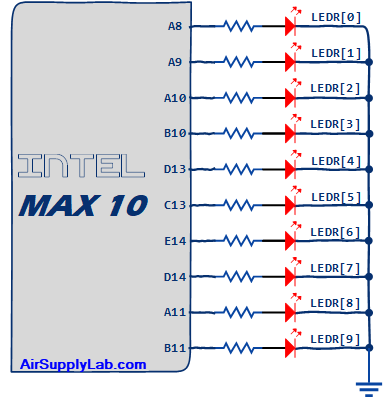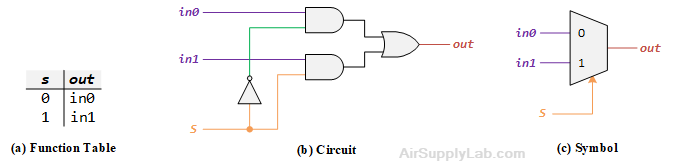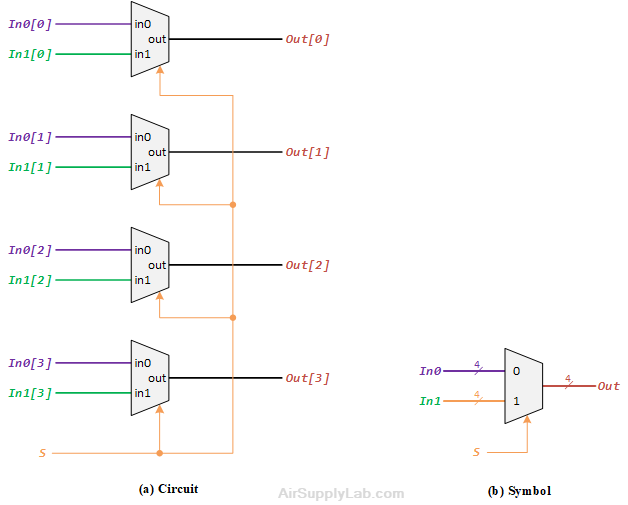# FPGA Lab 01: Switches, LEDs, and Multiplexers

## Objectives

The purpose of this exercise is to learn how to connect simple input and output devices to an FPGA chip and implement a circuit that uses these devices. We will use the switches on the DE10-Lite board as inputs to the circuit. We will use light-emitting diodes (LEDs) as output devices.

## Background Information

### DE10-Lite FPGA Board Switches and LEDs

The Intel DE10-Lite FPGA board has ten switches and LEDs. The connections between the switches and LEDs are shown in the following Figures:### Multiplexers

In digital circuits, a multiplexer (or MUX) is a device that selects one of several digital input signals and forwards the selected input into a single line. A multiplexer of 2n inputs has n select lines, which are used to select which input line to send to the output.

• A 2n-to-1 multiplexer sends one of the 2n input lines to a single output line.
• A multiplexer has two sets of inputs:
• 2n data input lines
• n select lines to pick one of the 2n data inputs
• The mux output is a single bit, which is one of the 2n data inputs.

Figure 2 (b) shows a sum-of-products circuit that implements a 2-to-1 multiplexer with a select input s. If s = 0, the output of the multiplexer (out) is equal to the input in0; if s = 1, the output is equal to in1. Part (a) of the figure gives a function table for this multiplexer, and part (c) shows its circuit symbol.Figure 2: A 2-to-1 Multiplexer

Write a Verilog module that includes four assignment statements like the one shown above to describe the circuit given in Figure 3 (a). This circuit has two four-bit inputs, In0 and In1, and produces the four-bit output Out. If S = 0 then Out = In0, while if S = 1 then Out = In1. We refer to this circuit as a four-bit wide 2-to-1 multiplexer, and its circuit symbol shows in Figure 3(b), in which In0, In1, and Out depict as four-bit wires.Figure 3: A four-bit wide 2-to-1 Multiplexer